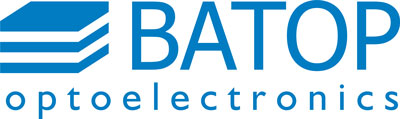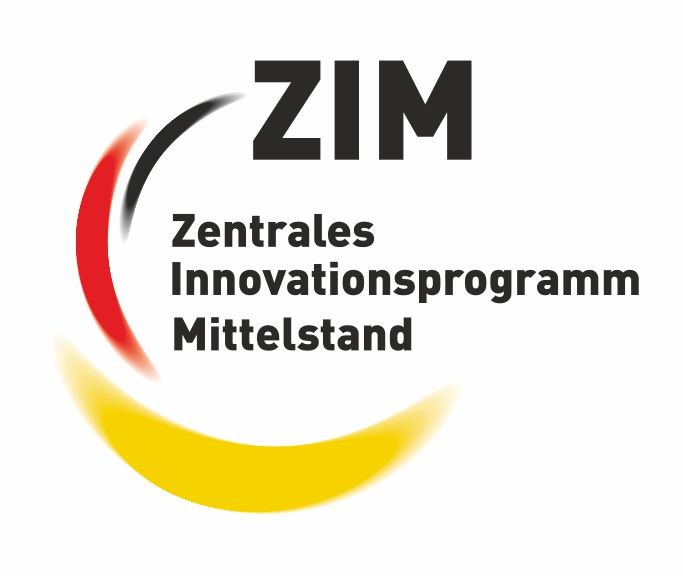#### SOC - Saturable Output coupler λ = 1040 nm

• see flyer "SOC - saturable output coupler" (pdf)

##### Attention:   SOC Order Information
Part-No description: SOC-λ-A-T-τ-x
Please select the main parameters from the tables below.
• λ - Laser wavelength
for example: 1040 nm
• A - Low intensity absorption
for example: 4 %
• T - Low intensity transmission
for example: 2 %
• τ - Absorber relaxation time
for example: 1 ps
Please select the mounting option from the box "Mounting condition x & price" below.
For Example, if you have choosen x=5.0-25.4g, mounting is as follows:
• Chip size: 5 mm x 5 mm
• Heat sink: 25.4 mm diameter with 4 mm center hole
• Mounting type: glued
• Position: center
Example:   SOC-1040-2-3-1ps-5.0-25.4g
• Laser wavelength: 1040 nm
• Absorption: 2 %
• Transmission: 3 %
• Relaxation time: 1 ps
• Chip size: 5 mm x 5 mm
• Glued on a copper heat sink with 25.4 mm diameter and 4 mm center hole

Product list SOC, λ = 1030 nm

• SOC-1030-0.5-3-1ps-x

• Wavelength λ = 1030 nm
• Absorptance A = 0.5 %
• Transmittance T = 3 %
• Relaxation time τ = 1 ps
Data sheet (pdf)
Mounting & price table
Order formThis work has been supported by the “Federal Ministry of Economics and Technology” program ZIM grant No. ZF4592301DF8.Product list SOC, λ = 1040 nm

• SOC-1040-2-3-1ps-x

• Wavelength λ = 1040 nm
• Absorptance A = 2 %
• Transmittance T = 3 %
• Relaxation time τ = 1 ps
Data sheet (pdf)
Mounting & price table
Order form

• SOC-1040-3-0.4-1ps-x

• Wavelength λ = 1040 nm
• Absorptance A = 3 %
• Transmittance T = 0.4 %
• Relaxation time τ = 1 ps
Data sheet (pdf)
Mounting & price table
Order form

• SOC-1040-3-4-1ps-x

• Wavelength λ = 1040 nm
• Absorptance A = 3 %
• Transmittance T = 4 %
• Relaxation time τ = 1 ps
Data sheet (pdf)
Mounting & price table
Order form

• SOC-1040-5-5-1ps-x

• Wavelength λ = 1040 nm
• Absorptance A = 5 %
• Transmittance T = 5 %
• Relaxation time τ = 1 ps
Data sheet (pdf)
Mounting & price table
Order form

• SOC-1040-6-0.5-1ps-x

• Wavelength λ = 1040 nm
• Absorptance A = 6 %
• Transmittance T = 0.5 %
• Relaxation time τ = 1 ps
Data sheet (pdf)
Mounting & price table
Order form

• SOC-1040-6-2-1ps-x

• Wavelength λ = 1040 nm
• Absorptance A = 6 %
• Transmittance T = 2 %
• Relaxation time τ = 1 ps
Data sheet (pdf)
Mounting & price table
Order form

• SOC-1040-7-2-1ps-x

• Wavelength λ = 1040 nm
• Absorptance A = 7 %
• Transmittance T = 2 %
• Relaxation time τ = 1 ps
Data sheet (pdf)
Mounting & price table
Order form

• SOC-1040-8-0.6-1ps-x

• Wavelength λ = 1040 nm
• Absorptance A = 8 %
• Transmittance T = 0.6 %
• Relaxation time τ = 1 ps
Data sheet (pdf)
Mounting & price table
Order form

• SOC-1040-8-6-15ps-x

• Wavelength λ = 1040 nm
• Absorptance A = 8 %
• Transmittance T = 6 %
• Relaxation time τ = 15 ps
Data sheet (pdf)
Mounting & price table
Order form

• SOC-1040-9-9-1ps-x

• Wavelength λ = 1040 nm
• Absorptance A = 9 %
• Transmittance T = 9 %
• Relaxation time τ = 1 ps
Data sheet (pdf)
Mounting & price table
Order form

• SOC-1040-11-3-1ps-x

• Wavelength λ = 1040 nm
• Absorptance A = 11 %
• Transmittance T = 3 %
• Relaxation time τ = 1 ps
Data sheet (pdf)
Mounting & price table
Order form

• SOC-1040-14-10-15ps-x

• Wavelength λ = 1040 nm
• Absorptance A = 14 %
• Transmittance T = 10 %
• Relaxation time τ = 15 ps
Data sheet (pdf)
Mounting & price table
Order form

• SOC-1040-17-5-1ps-x

• Wavelength λ = 1040 nm
• Absorptance A = 17 %
• Transmittance T = 5 %
• Relaxation time τ = 1 ps
Data sheet (pdf)
Mounting & price table
Order form

• SOC-1040-24-19-15ps-x

• Wavelength λ = 1040 nm
• Absorptance A = 24 %
• Transmittance T = 19 %
• Relaxation time τ = 15 ps
Data sheet (pdf)
Mounting & price table
Order form

Mounting condition x & price

• x = 5.0-0

• Single chip, unmounted
• Chip area 5.0 mm x 5.0 mm
• Chip thickness 625 µm
Data sheet (pdf)
• Price: 624.- €/pc

• x = 5.0-12.7g

• Chip area 5.0 mm x 5.0 mm
• Glued on a copper heat sink with 12.7 mm diameter and 4 mm center hole
Data sheet (pdf)
• Price: 702.- €/pc

• x = 5.0-25.4g

• Chip area 5.0 mm x 5.0 mm
• Glued on a copper heat sink with 25.4 mm diameter and 4 mm center hole
Data sheet (pdf)
• Price: 702.- €/pc

• x = FC

• SOC chip mounted on a single mode fiber cable with FC/PC connector
• free space output beam
Data sheet (pdf)
• Price: 871.- €/pc